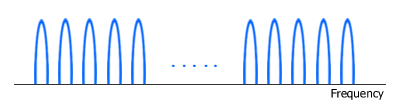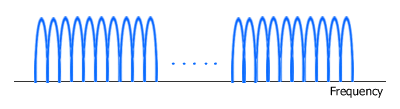#### Frequency Division Multiplexing (FDM)

WiMAX air interface is based on OFDM/OFDMA physical layer (PHY). To understand how OFDM and OFDMA work, it is useful to start with its "mother" namely FDM (Frequency Division Multiplexing).Picture. Frequency Division Multiplexing (FDM)
Spacing is put between two adjacent sub-carriers.

In FDM system, signals from multiple transmitters are transmitted simultaneously (at the same time slot) over multiple frequencies. Each frequency range (sub-carrier) is modulated separately by different data stream and a spacing (guard band) is placed between sub-carriers to avoid signal overlap.

#### Orthogonal Frequency Division Multiplexing (OFDM)Picture. Orthogonal Frequency Division Multiplexing (OFDM)
Sub-carriers are closely spaced until overlap.

Like FDM, OFDM also uses multiple sub-carriers but the sub-carriers are closely spaced to each other without causing interference, removing guard bands between adjacent sub-carriers. This is possible because the frequencies (sub-carriers) are orthogonal, meaning the peak of one sub-carrier coincides with the null of an adjacent sub-carrier.

In an OFDM system, a very high rate data stream is divided into multiple parallel low rate data streams. Each smaller data stream is then mapped to individual data sub-carrier and modulated using some sorts of PSK (Phase Shift Keying) or QAM (Quadrature Amplitude Modulation). i.e. BPSK, QPSK, 16-QAM, 64-QAM.

OFDM needs less bandwidth than FDM to carry the same amount of information which translates to higher spectral efficiency. Besides a high spectral efficiency, an OFDM system such as WiMAX is more resilient in NLOS environment. It can efficiently overcome interference and frequency-selective fading caused by multipath because equalizing is done on a subset of sub-carriers instead of a single broader carrier. The effect of ISI (Inter Symbol Interference) is suppressed by virtue of a longer symbol period of the parallel OFDM sub-carriers than a single carrier system and the use of a cyclic prefix (CP).

 Back Next